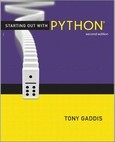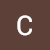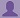SHARE
HELP

To start with, Sr2Jr’s first step is to reduce the expenses related to education. To achieve this goal Sr2Jr organized the textbook’s question and answers. Sr2Jr is community based and need your support to fill the question and answers. The question and answers posted will be available free of cost to all.# Starting Out With Python

Authors:
Exercise:
Chapter:
Introduction To Computers And Programming
Edition:
2
ISBN:
9780132576376
Question:
2
 Previous Next

###Question

What number does a bit that is turned on represent? What number does a bit that is turned off represent?

###Answer

In computer systems, a bit that is turned on represents the number 1. A bit that is turned off represents the number 0. This relates to the binary numbering system. In the binary numbering system or binary system, all numeric values are written as sequences of 0s and 1s.

An example of a binary number is written as follows

100011101

### Discussions

Post the discussion to improve the above solution.